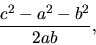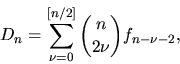Next: PLENARY SPEAKERS / CONFÉRENCIERS Up: PUBLIC LECTURE / CONFÉRENCE Previous: PUBLIC LECTURE / CONFÉRENCE

## H. S. M. Coxeter - The Descartes circle theorem and Fibonacci numbers

 H. S. M. COXETER, Department of Mathematics, University of Toronto, Toronto, Ontario  M5S 3G3, Canada The Descartes circle theorem and Fibonacci numbers

The numerical distance between two circles in the Euclidean plane is defined to be the numberwhere a and b are their radii while c is the ordinary distance between their centres. An infinite sequence of circles is defined to be loxodromic if every four consecutive members are mutually tangent. Dn denotes the numerical distance between the mth and (m+n)-th circles (the same for all m). Obviously D-n = Dn. Since the numerical distance is -1 when a=b and c=0 so that the two circles coincide, D0 = -1. Since it is 1 when a+b = c so that the circles are externally tangent, D1 = D2 = D3 = 1. Any number of further values of Dn can be determined successively by the recurrence equation

Dm + Dm+4 = 2(Dm+1 + Dm+2 + Dm+3) .

There is also an explicit formulain terms of binomial coefficients and Fibonacci numbers.Next: PLENARY SPEAKERS / CONFÉRENCIERS Up: PUBLIC LECTURE / CONFÉRENCE Previous: PUBLIC LECTURE / CONFÉRENCE